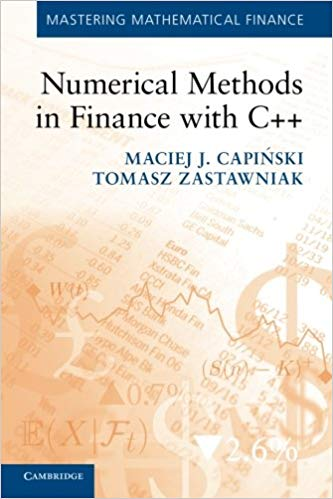# Binomial Pricing Model¶

### (Go to Quant Lab)¶

#### Source: Numerical Methods in Finance with C++¶

© Maciej J. Capinski, Tomasz ZastawniakInitially import all the modules we will be using for our notebook

In :
import math
import numpy as np
import numpy.random as npr
import sys
import os


## 1.1 Creating Main Program¶

In :
if __name__== "__main__":
print("Hi there")
input("Provide a character: ")

Hi there
Provide a character: 1


## 1.2 Entering Data¶

In :
if __name__== "__main__":
# Get input parameters S0, U, D, R

# make sure that 0< S0, -1 < D < U, -1 < R

# checking arbitrage condition

print("Input data checked")
print("There is no arbitrage\n")

# compute risk-neutral probability

# compute stock price at node n=3,i=2
n = 3; i = 2
print("n = ", n)
print("i = ", i)

Enter S_0: 1
Enter U: 2
Enter D: -0.5
Enter R: 0
Input data checked
There is no arbitrage

q =  0.2
n =  3
i =  2
S(n,i) =  4.5


## 1.3 Functions¶

In :
# computing risk-neutral probability
def RiskNeutProb(U, D, R):

# computing the stock price at node n,i
def S(S0, U, D, n, i):
'''
Returns the stock price at S(n, i)
'''

def isValidInput(S0, U, D, R):
'''
Returns: This function should return zero if any condition is violated, returns 1 otherwise
'''

# making sure that 0<S0, -1<D<U, -1<R

# checking for arbitrage

# inputting, displaying and checking model data
def GetInputData():
'''
This function accepts user input and updates the variables on your computer locally
'''
#entering data
params = ("S0", "U", "D", "R")
S0, U, D, R = [float(input("Enter %s: " % (var))) for var in params]

if not isValidInput(S0, U, D, R):
return 0

print("Input data checked")
print("There is no arbitrage\n")
d = locals().copy()
return d

q =  0.13043478260869565
Enter S0: 3
Enter U: 2
Enter D: -0.2
Enter R: 0
Input data checked
There is no arbitrage

n =  3
i =  2
S(n,i) =  21.6


If your functions above are correct, then run this program

In [ ]:
# If your functions above are correct, then run this program
if __name__== "__main__":
# compute risk-neutral probability
print("q = ", RiskNeutProb(U, D, R))

output = GetInputData()
if output == 0:
sys.exit()

# Update our parameters
locals().update(output)

# compute stock price at node n=3,i=2
n = 3; i = 2
print("n = ", n)
print("i = ", i)
print("S(n,i) = ", S(S0,U,D,n,i))


## 1.4 Option Pricing Definitions¶

In [ ]:
def PriceByCRR(self, Model, N=3):
'''
Implement European style option pricing
'''

def PriceBySnell(self, Model, N=3):
'''
Implement American style option pricing
'''


## 2.0 Object Oriented European and American¶

In :
# Binomial Model (Parent class) ---------------------------------------------------
class BinModel:
'''
Parent class for a generic binomial model which holds the model parameters
'''

# European Option class ------------------------------------------------------------------
class EurOption():

def PriceByCRR(self, Model, N=3):
'''
Returns the option price at time 0 if the option is European style
'''

# American Option class
class AmOption():
def PriceBySnell(self, Model, N=3):
'''
Returns the option price at time 0 if the option is American style
'''

# Payoff Classes ----------------------------------------------------------------------------
class Call(EurOption, AmOption):
'''
Class for Call option payoff
'''

class Put(EurOption, AmOption):
'''
Class for Put option payoff
'''

Out:
0.4482083442210392
In [ ]:
# Testing with parameters
model = BinModel(S0=10, U=0.2, D=-0.1, R=0.1)
Option1 = Call(K=22)
Option1.PriceByCRR(model, N=6)


## Exercises:¶

1. Modify the PriceByCRR() function in EurOption Class to compute the time $0$ price of a European option using the Cox–Ross–Rubinstein (CRR) formula: $$H(0)=\frac{1}{(1+R)^{N}}\sum_{i=0}^{N}\frac{N!}{i!(N-i)!}q^{i}(1-q)^{N-i}h(S(N,I))$$
1. The payoff of a digital call with strike price $K$ is: $$h^{digit\thinspace call}(z)=\begin{cases} 1 & \textrm{if }K<z,\\ 0 & \textrm{otherwise.} \end{cases}$$ Include the ability to price digital calls in the program developed in the present section by adding the new payoff Class DigitCall just as was done for calls and puts.
1. Add the ability to price bull spreads and bear spreads by introducing new subclasses BullSpread and BearSpread of the EurOption and AmOption classes defined in our current program. The payoffs of a bull spread and a bear spread, which depend on two parameters $K1 < K2$, are given by: $$h^{bull}(z)=\begin{cases} 0 & \textrm{if }z\leq K_{1},\\ z-K_{1} & \textrm{if }K_{1}<z<K_{2}\\ K_{2}-K_{1} & \textrm{if }K_{2}\leq z, \end{cases}$$ and $$h^{bear}(z)=\begin{cases} K_{2}-K_{1} & \textrm{if }z\leq K_{1},\\ K_{2}-z & \textrm{if }K_{1}<z<K_{2}\\ 0 & \textrm{if }K_{2}<z, \end{cases}$$
1. Consider the fixed-strike Asian call option with process, $$A_{N}=\frac{1}{N}\sum_{k=0}^{N-1}S(k)$$ and payoff, $$C(N)=max\{A_{N},0\}$$ Add a new Class AsianOption to price an Asian style Call Option.